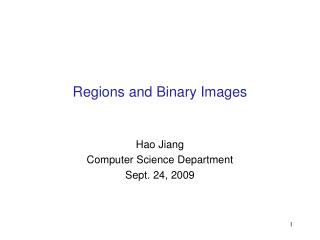# Regions and Binary Images - PowerPoint PPT PresentationDownload PresentationRegions and Binary Images

Regions and Binary ImagesDownload Presentation## Regions and Binary Images

- - - - - - - - - - - - - - - - - - - - - - - - - - - E N D - - - - - - - - - - - - - - - - - - - - - - - - - - -
##### Presentation Transcript

1. Regions and Binary Images Hao Jiang Computer Science Department Sept. 24, 2009

2. Figure Ground Separation

3. Brightness Thresholding

4. Thresholding • Given a grayscale image or an intermediate matrix  threshold to create a binary output. Example: background subtraction - = Looking for pixels that differ significantly from the “empty” background. Slide from Kristen Grauman fg_pix = find(diff > t);

5. Thresholding • Given a grayscale image or an intermediate matrix  threshold to create a binary output. Example: color-based detection fg_pix = find(hue > t1 & hue < t2); Looking for pixels within a certain hue range. Slide from Kristen Grauman

6. More Binary Images Slide from Kristen Grauman

7. Issues • How to demarcate multiple regions of interest? • Count objects • Compute further features per object • What to do with “noisy” binary outputs? • Holes • Extra small fragments Slide from Kristen Grauman

8. Find Connected Regions

9. Find Connected Regions Our target in this image is the largest blob.

10. Connected Components • Identify distinct regions of “connected pixels” Shapiro and Stockman

11. Pixel Neighbors 4 neighboring pixels of the blue pixel

12. Pixel Neighbors 8 neighboring pixels of the blue pixel

13. Recursive Method label = 2 for i = 1 to rows for j = 1 to cols if I(i, j) == 1 labelConnectedRegion(i, j, label) label ++; end end

14. Recursive Method function labelConnectedRegion(int i, int j, int label) if (i,j) is labeled or background or out of boundary return I(i,j)=label for (m,n) belongs to neighbors of (i,j) labelConnectedRegion(m,n,label) end

15. Two Pass Method • We check each pixel from left to right and up to bottom • If a pixel has no left and up foreground neighbor, we assign a new label to the pixel • If a pixel has only one left or up foreground neighbor, we assign the label of the neighbor to the pixel • If a pixel has both left and up foreground neighbors, and their labels are the same, we assign the label of the neighbor to the pixel • If a pixel has both left and up foreground neighbors, and their labels are the different, we assign the label of the left neighbor to the pixel and use the up-left label pair to update the equivalency table. • We go through another pass to replace the labels to corresponding labels in the equivalency table

16. Example Image Label equivalence table

17. Example Image Label equivalence table

18. Example Image Label equivalence table

19. Example Image Label equivalence table

20. Example Image Label equivalence table

21. Example Image Label equivalence table

22. Example Image Label equivalence table

23. Example Image Label equivalence table

24. Example Image Label equivalence table

25. Example (2,3) Image Label equivalence table

26. Example Image Label equivalence table

27. Example Image Label equivalence table

28. Example Image Label equivalence table

29. Example Image Label equivalence table

30. Example Image Label equivalence table

31. Example (5,2) Image Label equivalence table

32. Example Image Label equivalence table

33. Example Image Label equivalence table

34. Example Image Label equivalence table

35. Example Image Label equivalence table

36. Example Image Label equivalence table

37. Example Image Label equivalence table

38. Example (5,2) Image Label equivalence table

39. Example Image Label equivalence table

40. Example Image Label equivalence table

41. Morphology Operations • Define At = { p + t | p is a point in A} • Erosion T = { t | At belongs to S} A Erosion(S, A) S

42. Morphology Operations • Define At = { p + t | p is a point in A} • Erosion T = { t | At belongs to S} What if A is S

43. Morphology Operations • Define At = { p + t | p is a point in A} • Dilation T = Union of At and S for all t in S What if A is Erosion(S, A) S

44. Morphology Operations • Define At = { p + t | p is a point in A} • Dilation T = Union of At and S for all t in S What if A is S

45. Morphology Operations • Define At = { p + t | p is a point in A} • Dilation T = Union of At and S for all t in S What if A is

46. Opening • Erode, then dilate • Remove small objects, keep original shape Before opening After opening Slide from Kristen Grauman

47. Closing • Dilate, then erode • Fill holes, but keep original shape Before closing After closing Slide from Kristen Grauman

48. Morphology Operators on Grayscale Images • Dilation and erosion typically performed on binary images. • If image is grayscale: for dilation take the neighborhood max, for erosion take the min. eroded original dilated Slide from Kristen Grauman

49. Matlab • Create structure element se = strel(‘disk’, radius); • Erosion imerode(image, se); • Dilation imdilate(image, se); • Opening imopen(image, se); • Closing imclose(image, se); • More possibilities bwmorph(image, ‘skel’);

50. Figure Ground Separation